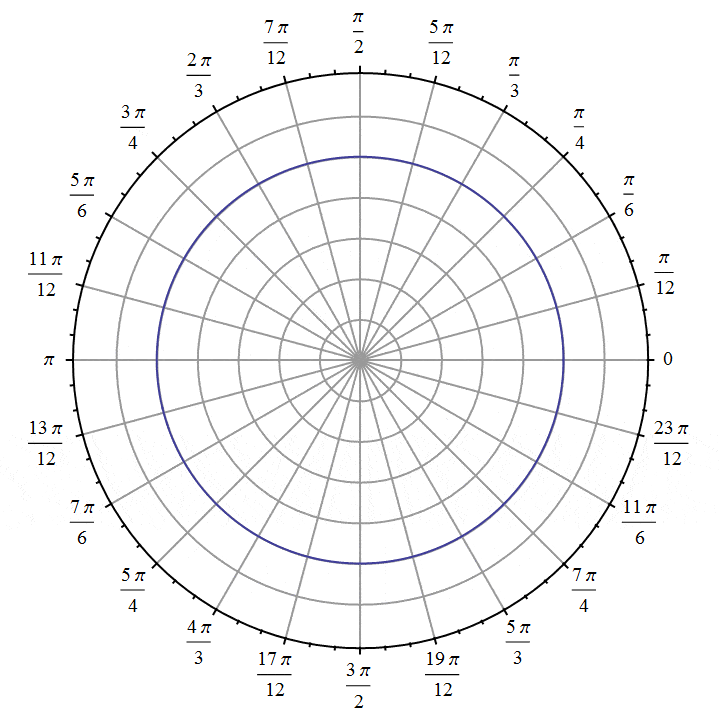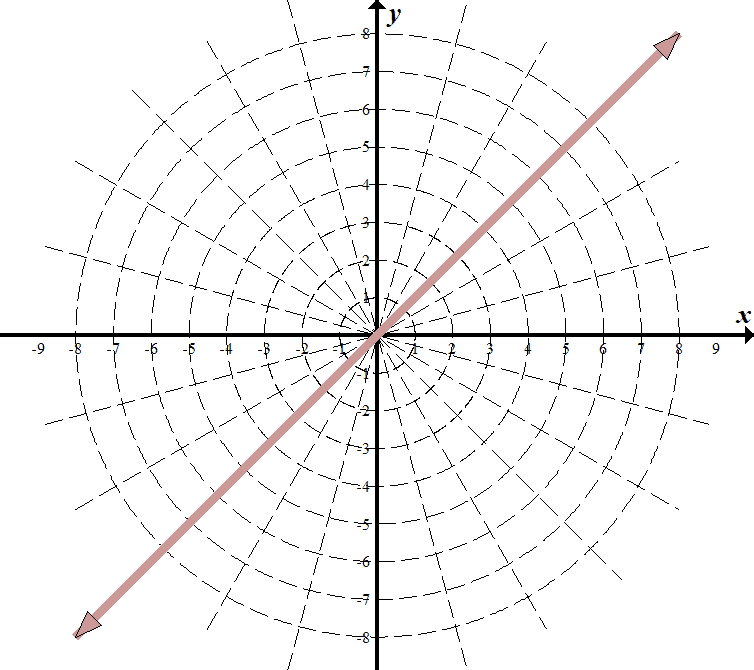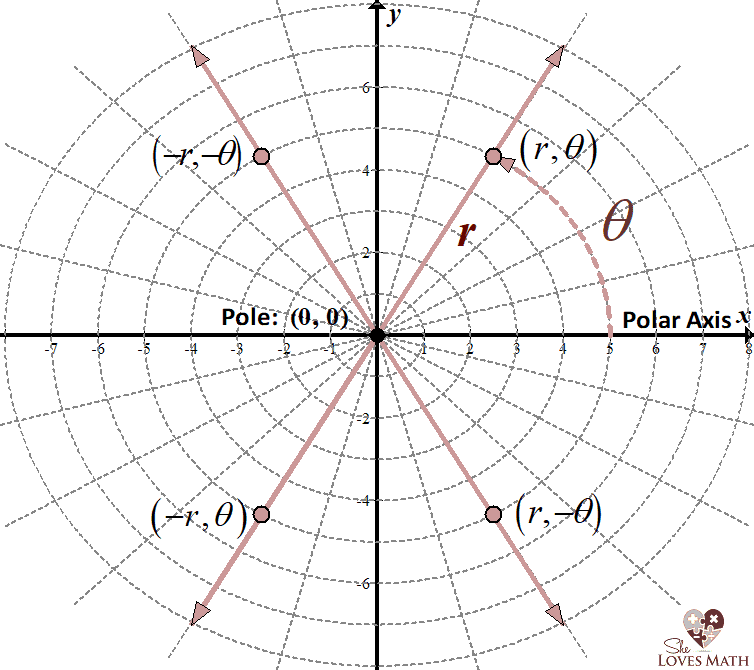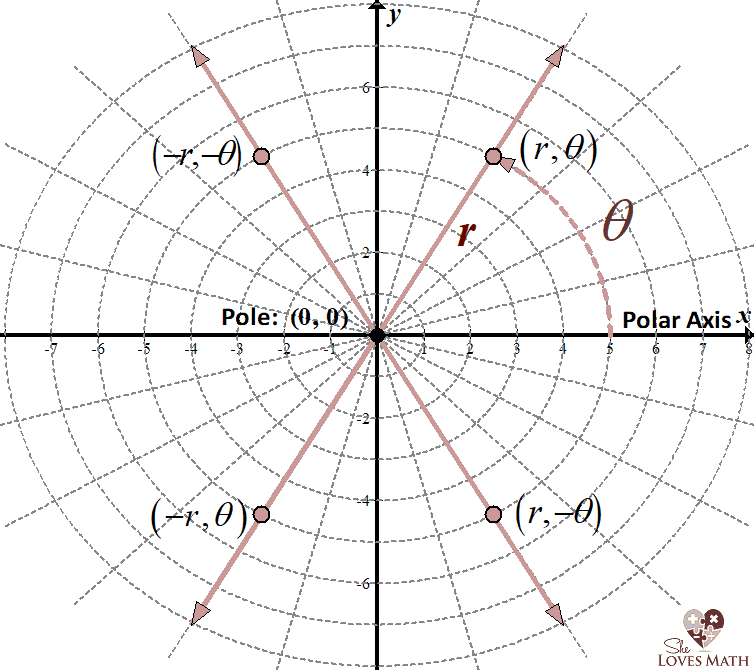# Graphing θ=π/4 on a Polar Coordinate System

Homework Statement:
Graph ##θ=\frac{π}{4}## on a Polar Coordinate System.
Relevant Equations:
Why does the line go into the opposite quadrant as well?
When you graph something like ##θ=\frac{π}{4}## on a Polar Coordinate System:
Why does the line go into the opposite quadrant as well?
I can intuitively understand why it is in the first quadrant: ##θ = 45°## there and so all possible values of ##r## would apply there, giving you a straight line headed outwards at an angle of ##45°##.
So, why does the line go into the opposite quadrant as well?
Isn't ##r## always positive? Or, is that something we define beforehand as being either positive or negative?•member 587159

QuantumQuest
Gold Member
In polar coordinates, the angle that corresponds to a certain point is not unique. For example, let's say that we have a point, at a distance of 2 units from the origin on the radius with ##θ=30^0##. It has polar coordinates ##r=2,θ=30^0##. It has also coordinates ##r=2,θ=−330^0## and ##r=2,θ=390^0##. So, it depends on what direction we traverse the circle - as in Trigonometry an angle ##θ## is positive when it runs counter-clockwise, and how many times we traverse the circle.

EDIT: Also, the radius ##r##, can be negative. So, we talk about an oriented distance. For instance, the radius with ##θ=30^0## and the one with ##θ=210^0## form a straight line which passes through the origin. The point, say, ##P(2,210^0)## which is at a distance of ##2## units from the origin on the radius with ##θ=210^0##, has polar coordinates ##r=2,θ=210^0##. We can reach this point if we go in the positive direction ##210^0## and walk ##2## units forward. We can also reach the same point if we turn ##30^0## in the positive direction and walk ##2## units backwards. The turns we take are with reference to the initial radius (##\theta = 0##), given that we are at the origin (##O##). So, the previous point we talked about, has also polar coordinates ##r = -2, \theta = 30^0##.

Last edited:
•lightlightsup
In polar coordinates, the angle that corresponds to a certain point is not unique. For example, let's say that we have a point, at a distance of 2 units from the origin on the radius with ##\theta = 30^0##. It has polar coordinates ##r = 2, \theta = 30^0##. It has also coordinates ##r = 2, \theta = -330^0## and ##r = 2, \theta = 390^0##. So, it depends on what direction we traverse the circle - as in Trigonometry an angle ##\theta## is positive when it runs counter-clockwise, and how many times we traverse the circle.
But, in my question above, isn't the opposite angle ##225°## and not some other angle equivalent to ##45°## (like ##-315°## or ##405°##)?
Am I stuck thinking in terms of the unit circle definition?

member 587159
If you don't allow negative radius, I think you are right. Then it should be a half line in the first quadrant. Changing the angle to an equivalent doesn't change this.

•lightlightsup
QuantumQuest
Gold Member
•lightlightsup
EDIT: Also, the radius rr, can be negative. So, we talk about an oriented distance. For instance, the radius with θ=300θ=30^0 and θ=2100θ=210^0 form a straight line which passes through the origin. The point P(2,2100)P(2,210^0) which is at a distance, say, of 2 units from the origin on the radius with θ=2100θ=210^0, has polar coordinates r=2,θ=2100r=2,θ=210^0. We can reach this point if we go in the positive direction 2100210^0 and walk 2 units forward. We can also reach the same point if we turn 30030^0 in the positive direction and walk 2 units backwards. The turns we take are with reference to the initial radius (θ=0\theta = 0), given that we are at the origin (OO). So, the previous point we talked about has also polar coordinates r=−2,θ=300r = -2, \theta = 30^0.
So, it's kind of like ##-\hat{r}##?
But, in the formal/mathematical case, we would explicitly have to state what ##r## is allowed to be (like ##-r##)?

So, the way I'm understanding the sign on ##θ## is that it is defined independently in each quadrant based on whether it is counterclockwise (+) or clockwise (-):
For example: ##+30°## would occur in Quadrants I and III because it would be ##30°## counterclockwise from the Polar x-Axis there. ##-30°## would occur in Quadrants II and IV because it would be clockwise from the Polar x-Axis there.
So, the mystery remains: how is ##r##'s sign defined? Left or Right from the Polar y-Axis?QuantumQuest
Gold Member
So, the mystery remains: how is ##r##'s sign defined?

There is no mystery. It's all a matter of convention. In math, conventions are there in order to talk about the same things in the same way. For the angle ##\theta## we use the positive sign (##+##) when we traverse the circle counter-clockwise and negative sign (##-##) when we traverse it clockwise. For the radius ##r## we use positive sign when we move from the origin to the outer side of the circle(s) on a certain radius and negative sign in the opposite case.

•lightlightsup
So, the convention is that ##r## is ##+## when it is in the ##+## direction of the Polar x-Axis.
And, ##θ##'s signage is determined by clockwise/counterclockwise angling relative to the Polar x-Axis.
Does that seem like a correct interpretation?
I can't think of any other explanation for why, by convention, ##(−r,−θ)## is in Quadrant II and ##(r,−θ)## is in Quadrant IV.
Another convention I've seen is that ##r## is always pointing outwards based on how the ##θ (+ or -)## guides it. But, it can point it in the opposite direction with a ##-## before the ##r##. This is the correct answer, I think. Determine the angle first, then figure out which direction you're heading in.This is why when you graph ##θ=\frac{π}{4}##, you include it in Quadrants I and III: you are considering all values of ##r## for which ##θ=\frac{π}{4}##?

Last edited:
QuantumQuest
Gold Member
So, the convention is that ##r## is ##+## when it is in the ##+## direction of the Polar x-Axis.
And, ##θ##'s signage is determined by clockwise/counterclockwise angling relative to the Polar x-Axis.
Does that seem like a correct interpretation?
I can't think of any other explanation for why, by convention, ##(−r,−θ)## is in Quadrant II and ##(r,−θ)## is in Quadrant IV.
Another convention I've seen is that ##r## is always pointing outwards based on how the ##θ (+ or -)## guides it. But, it can point it in the opposite direction with a ##-## before the ##r##. This is the correct answer, I think. Determine the angle first, then figure out which direction you're heading in.
View attachment 255485
This is why when you graph ##θ=\frac{π}{4}##, you include it in Quadrants I and III: you are considering all values of ##r## for which ##θ=\frac{π}{4}##?

The whole point is the reference you use. In my example in post #2 I talked about the different polar coordinates of the same point. In the diagram you give, there is the same angle ##\theta## with different sign per case. So, in the first quadrant as per your diagram, we have obviously ##(r,\theta)##. In the second quadrant the angle is ##-\theta## as it is seen clockwise beginning from the negative ##-x## axis. Then, the radius is ##-r## with respect to the direction we move. In the same manner we find the signs of ##r## and ##\theta## in the rest two quadrants.

Last edited:
•lightlightsup
Think about the equation y = 2 in a rectangular coordinate system. Why is this a line that goes both to the left and the right? Because x can be any real value and the statement is still true. It is the same for polar coordinates. If I say θ=π/4, then r can be any value, positive or negative.

•lightlightsup